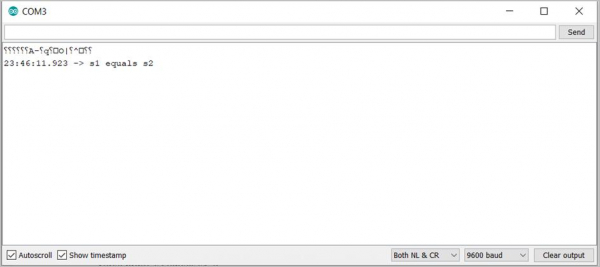# Check if two strings are equal or not in Arduino

In order to check if two strings are equal, the .equals() function can be used. This returns a Boolean. True if both the strings are equal, else false. An example code is given below −

## Example

void setup() {
// put your setup code here, to run once:
Serial.begin(9600);
Serial.println();
String s1 = "Hello";
String s2 = "Hello";
String s3 = "World";
if(s1.equals(s2)){
Serial.print("s1 equals s2");
}
if(s1.equals(s3)){
Serial.print("s1 equals s3");
}
if(s2.equals(s3)){
Serial.print("s2 equals s3");
}
}
void loop() {
// put your main code here, to run repeatedly:
}

As you would have guessed, only "s1 equals s2" gets printed on the Serial Monitor.

## Output